### Integrating the stresses in beams to obtain the section forces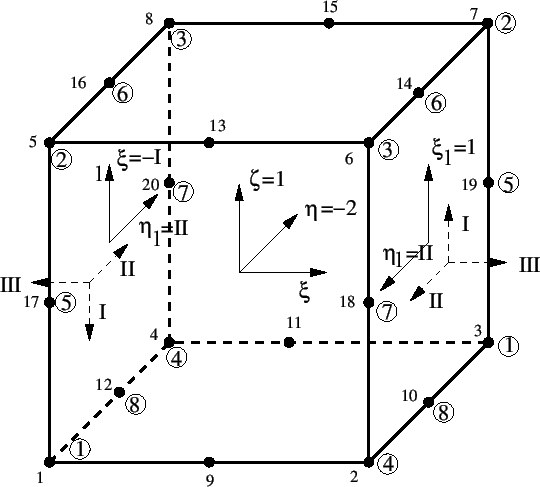In beam elements the section forces can be requested at the end nodes. To this end the stresses in the expanded faces at the end nodes are integrated. How this is done can be explained by looking at Figure 161.

The stresses in the expanded element are at first determined in the integration points (e.g. the Gauss-Kronrod points, cf. Figure 76). Then, they are expanded to the nodes of the element. Consequently, the stresses are available at all 20 nodes of the element in Figure 161. In order to obtain the section force the following local coordinate systems are introduced:

• The local element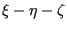-system.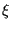is along the axis of the beam (from the first node of the element to the last node),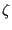is along the user-defined 1-direction of the beam,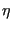corresponds to the minus 2-direction. The 2-direction is defined such that-1-2 corresponds to a positive axis system.
• On the positive face of the beam element (the face corresponding to the last end node of the beam definition, i.e.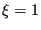) the positive direction for the section forces, which is denoted by I, II and III in Figure 161 corresponds to the element 1-direction, 2-direction and.
• On the negative face of the beam element the positive direction for the section forces, denoted by I, II and III points in the other direction of the corresponding axes on the positive face (action=reaction).
• The local node numbering within the positive face is labeled by 1 to 8 in small circles and corresponds to a local coordinate system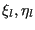=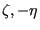coinciding with the system I-II.
• The local node numbering within the negative face is also labeled by 1 to 8 in small circles and corresponds to a local coordinate system= -I,II =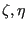.

The system I-II-III in the faces denotes the positive direction of the section forces. The location of the integration points in the correspondingsystem is obtained from the local element coordinate systemthrough the above face-dependent relationships.

In order to get the section forces the stresses are calculated in the integration points of the positive and negative face by interpolation from the stresses at the nodes belonging to the respective face. The integration point scheme depends on the beam section.